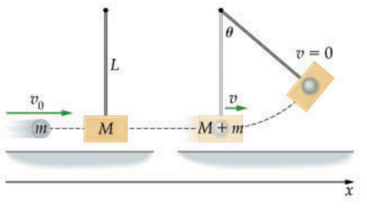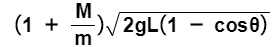# Problem: In a ballistic pendulum an object of mass m is fired with an initial speed v0 at a pendulum bob. The bob has a mass M, which is suspended by a rod of length L and negligible mass. After the collision, the pendulum and object stick together and swing to a maximum angular displacement θ as shown (Figure 1)The expression for the initial velocity v0 of the bullet in terms of m, M, L, θ, and the acceleration due to gravity, g is An experiment is done to compare the initial speed of bullets fired from different handguns: a 9.0 mm and a .44 caliber. The guns are fired into a 10-kg pendulum bob of length L. Assume that the 9.0-mm bullet has a mass of 6.0 g and the .44-caliber bullet has a mass of 12 g . If the 9.0-mm bullet causes the pendulum to swing to a maximum angular displacement of 4.3?and the .44-caliber bullet causes a displacement of 10.1? , find the ratio of the initial speed of the 9.0-mm bullet to the speed of the .44-caliber bullet, (v0)9.0/(v0)44.Express your answer numerically.

###### FREE Expert Solution

Veocity of the bullet before collision:

M = 10kg

m9.0 = 0.006 kg

m.44 = 0.012 kg

θ9.0 = 4.3°

θ.44 = 10.1°###### Problem Details

In a ballistic pendulum an object of mass m is fired with an initial speed v0 at a pendulum bob. The bob has a mass M, which is suspended by a rod of length L and negligible mass. After the collision, the pendulum and object stick together and swing to a maximum angular displacement θ as shown (Figure 1)The expression for the initial velocity v0 of the bullet in terms of m, M, L, θ, and the acceleration due to gravity, g isAn experiment is done to compare the initial speed of bullets fired from different handguns: a 9.0 mm and a .44 caliber. The guns are fired into a 10-kg pendulum bob of length L. Assume that the 9.0-mm bullet has a mass of 6.0 g and the .44-caliber bullet has a mass of 12 g . If the 9.0-mm bullet causes the pendulum to swing to a maximum angular displacement of 4.3?and the .44-caliber bullet causes a displacement of 10.1? , find the ratio of the initial speed of the 9.0-mm bullet to the speed of the .44-caliber bullet, (v0)9.0/(v0)44.